Courses

# Test: Physics- 4

## 45 Questions MCQ Test NEET Mock Test Series | Test: Physics- 4

Description
This mock test of Test: Physics- 4 for NEET helps you for every NEET entrance exam. This contains 45 Multiple Choice Questions for NEET Test: Physics- 4 (mcq) to study with solutions a complete question bank. The solved questions answers in this Test: Physics- 4 quiz give you a good mix of easy questions and tough questions. NEET students definitely take this Test: Physics- 4 exercise for a better result in the exam. You can find other Test: Physics- 4 extra questions, long questions & short questions for NEET on EduRev as well by searching above.
QUESTION: 1

### Figure shows some of the electric field lines corresponding to an electric field. The figure suggests that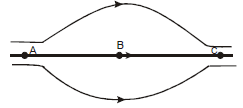Solution:
Electric field is stronger where electric field lines are closer as depicted in the figure the field lines are almost having same distance near point A and C thus they are equal and near point B they are far thus indicating weak field at B .
At A and C, electric lines are equally spaced and dense that's why EA = EC > EB
QUESTION: 2

Solution:
QUESTION: 3

### An electric dipole is placed in a uniform electric field.The net electric force on the dipole

Solution:
QUESTION: 4

The electric field in a region is directed outward and is proportional to the distance r from the origin.Taking the electric potential at the origin to be zero,

Solution:
QUESTION: 5

A charge Q is uniformly distributed over a large plastic plate. The electric field at a point P close to the centre of the plate is 10 V/m. If the plastic plate is replaced by a copper plate of the same geometrical dimensions and carrying the same charge Q, the electric field at the

point P will become

Solution:
QUESTION: 6

Electric charge are distributed in a small volume. The flux of the electric field through a spherical surface of radius 10cm surrounding the total charge is 25V-m The flux over a concentric sphere of radius 25cm will be

Solution:
QUESTION: 7

100V–m 4. A charge q is placed at the centre of the open end of a cylindrical vessel in figure. The flux of the electric field through the surface of the vessel is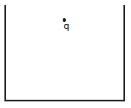Solution:
QUESTION: 8

Mark the correct options

Solution:
QUESTION: 9

Figure shows a closed surface which intersects a conducting sphere. If a positively charged is placed at the point P, the flux of the electric field through the closed surface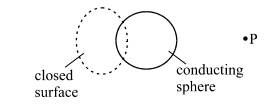Solution:
QUESTION: 10

Two capacitors each having capacitance C and breakdown voltage V are joined in series. The capacitance and the breakdown voltage of the combination will be

Solution:
QUESTION: 11
A dielectric slab is inserted between the plates of an isolated capacitor. The force between the plates will
Solution:
QUESTION: 12

Two metal spheres of capacitances  C& C2 carry some charges. They are put in contact and then separated. The final charges  Q& Q2 on them will satisfy

Solution:
QUESTION: 13

A dielectric slab is inserted between the plates of an isolated charged capacitor. Which of the following quantities will remain the same?

Solution:
QUESTION: 14
A parallel-plate capacitor is connected to a battery. A metal sheet of negligible thickness is placed between the plates. The sheet remains parallel to the plates of the capacitor
Solution:
QUESTION: 15

A uniform wire of resistance 50 Ω is cut into 5 equal parts. These parts are now connected in parallel. The equivalent resistance of the combination is

Solution:
QUESTION: 16

The net resistance of an ammeter should be small to ensure that

Solution:
QUESTION: 17

Consider a capacitor charging circuit. Let Qbe the charge given to the capacitor in a time interval of 10 ms and  Q2 be the charge given in the next time interval of of 10 ms. Let 10 μC charge be deposited in a time interval t1 and the next 10 μC charge is deposited in the next time interval t2. Then,

Solution:
QUESTION: 18
Which one of the following quantities do not change when a resistor connected to a battery is heated due to the current ?
Solution:
QUESTION: 19
The equivalent capacitance between the points A and B of five identical capacitors each of capacity C, will be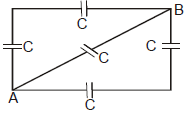Solution:
QUESTION: 20

Four condensers each of capacity 4mF are connected as shown in the figure. If VP - VQ = 15 V the energy stored in the system is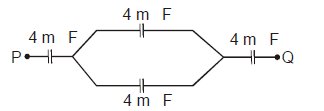Solution:
QUESTION: 21
If an electron is brought towards an another electron, the electric potential energy of the system
Solution:
QUESTION: 22
A hollow charged metal sphere has radius r. If the potential difference between its surface and a point at a distance 3r from the centre is V, then electric field intensity at a distance 3r is
Solution: FOR a sphere of charge Q and radius R∆V=(KQ/R) - (KQ/3R)=2KQ/3R Electric field=KQ/(3R)² =KQ/9R² =V/6R.
QUESTION: 23

If the separation between the plates of a capacitor is 5 mm, then the area of the plate of a 3 F parallel plate capacitor is

Solution:
QUESTION: 24

An insulated charged sphere of radius 5 cm has a potential of 10 V at the surface. The potential at the centre will be

Solution:
QUESTION: 25
A particle of mass 2 g and charge 1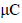is held at a distance of 1m from a fixed charge 1mC. If the particle is released it will be repelled. The speed of particle when it is at a distance of 10 m from the fixed charge is
Solution:
QUESTION: 26

Two materials having the dielectric constants K1 and K2, are filled between two parallel plates of a capacitor, which is shown in the figure. The capacity of the capacitor is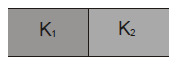Solution:
QUESTION: 27

Two point charges Q and –2Q are placed at some distance apart. If the electric field at the location of Q is E, the electric field at the location of –2Q will be

Solution:
QUESTION: 28

Shown in the figure is a distribution of charges. The flux of electric field due to these charges through the surface S is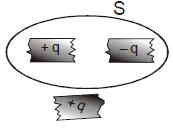Solution:
QUESTION: 29
Ten identical cells each of emf E and internal resistance r are connected in series to form a closed circuit. An ideal voltmeter connected across the three cells will read
Solution:
QUESTION: 30

What is the equivalent resistance between A and B in the given figure ?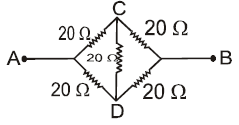Solution:
QUESTION: 31

A battery of emf 20V and internal resistance 6 Ω is connected to a resister as shown in figure. If the current in the circuit is 1A, the resistance of the resistor will be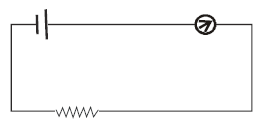Solution:
QUESTION: 32
Given a current carrying wire of non-uniform cross-section. Which one of the following is constant through out the length of wire
Solution:
QUESTION: 33

An electric bulb marked 40 W and 200 V, is used in a circuit of supply voltage 100 V. Its power is

Solution:
QUESTION: 34

A moving coil galvanometer is converted into an ammeter reading upto 0.03 A by connecting a shunt of resistance 4r across it and into ammeter upto 0.06 A, when a shunt of resistance r is used, what is the maximum current which can be sent through this galvanometer if no shunt is used

Solution:
QUESTION: 35

A galvanometer can withstand safely a maximum current of 5 mA. It is converted into voltmeter reading upto 20V by connecting in series an external resistance of 3.96 k Ω .The resistance of galvanometer is

Solution:
QUESTION: 36

Three resistors of 2 Ω , 3 Ω and 4 Ω  are connected as shown in the given diagram, then the equivalent resistance will be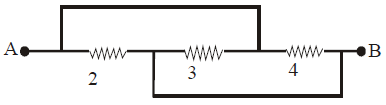Solution:
QUESTION: 37

Direction : Read the following question and choose

A. If both Assertion and Reason are correct and reason is the correct explanation of assertion

B. If both Assertion and Reason are true, but Reason is not correct explanation of the Assertion

C. If Assertion is true, but the Reason is false

D. If Assertion is false, but the Reason is true

E. If both assertion and reason are false

Assertion : The capacity of conductor, under given circumstances remains constant irrespective of the charge present on it

Reason: Capacity depends on size and shape of conductor and also on the medium between the plates.

Solution:
QUESTION: 38

Direction : Read the following question and choose

A. If both Assertion and Reason are correct and reason is the correct explanation of assertion

B. If both Assertion and Reason are true, but Reason is not correct explanation of the Assertion

C. If Assertion is true, but the Reason is false

D. If Assertion is false, but the Reason is true

E. If both assertion and reason are false

Assertion: A small metal ball is suspended in a uniform electric field with an insulated thread. If high energy X-ray beam falls on the ball, the ball will be deflected in the electric field.

Reason : X-rays emit photoelectrons and metal becomes negatively charged

Solution:
QUESTION: 39

Direction: Read the following question and choose

A. If both Assertion and Reason are correct and the reason is the correct explanation of assertion

B. If both Assertion and Reason are true, but Reason is not a correct explanation of the Assertion

C. If Assertion is true, but the Reason is false

D. If Assertion is false, but the Reason is true

E. If both assertion and reason are false

Assertion: If a point charge be rotated in a circle around a charge, the work done will be zero.

Reason: Work done is equal be dot product of force and displacement.

Solution:
QUESTION: 40

Direction : Read the following question and choose

A. If both Assertion and Reason are correct and reason is the correct explanation of assertion

B. If both Assertion and Reason are true, but Reason is not correct explanation of the Assertion

C. If Assertion is true, but the Reason is false

D. If Assertion is false, but the Reason is true

E. If both assertion and reason are false
Assertion: At a point in space, the electric field points towards north, In the region, surrounding this point the rate or change of potential will be zero along the east and west

Reason: Electric field due to a charge is the space around the charge in which some another charge experienced a force

Solution:
QUESTION: 41
Direction : Read the following question and choose
A. If both Assertion and Reason are correct and reason is the correct explanation of assertion
B. If both Assertion and Reason are true, but Reason is not correct explanation of the Assertion
C. If Assertion is true, but the Reason is false
D. If Assertion is false, but the Reason is true
E. If both assertion and reason are false

Assertion: When a dielectric medium is filled between the plates of a condenser, its capacitance increases.
Reason : The dielectric medium reduces the potential difference between the plates of the condenser
Solution:
QUESTION: 42

Direction : Read the following question and choose

A. If both Assertion and Reason are correct and reason is the correct explanation of assertion

B. If both Assertion and Reason are true, but Reason is not correct explanation of the Assertion

C. If Assertion is true, but the Reason is false

D. If Assertion is false, but the Reason is true

E0. If both assertion and reason are false

Assertion : At any junction of a network, algebraic sum of various currents is zero

Reason : Current cannot be stored.

Solution:
QUESTION: 43
Direction : Read the following question and choose
A. If both Assertion and Reason are correct and reason is the correct explanation of assertion
B. If both Assertion and Reason are true, but Reason is not correct explanation of the Assertion
C. If Assertion is true, but the Reason is false
D. If Assertion is false, but the Reason is true
E. If both assertion and reason are false

Assertion : Voltmeter is much better than a potentiometer for measuring emf of cell
Reason: A potentiometer draws no current while measuring emf of a cell
Solution:
QUESTION: 44
Direction : Read the following question and choose
A. If both Assertion and Reason are correct and reason is the correct explanation of assertion
B. If both Assertion and Reason are true, but Reason is not correct explanation of the Assertion
C. If Assertion is true, but the Reason is false
D. If Assertion is false, but the Reason is true
E. If both assertion and reason are false

Assertion: If the length of the conductor is doubled, the drift velocity will become half of the original value (keeping potential difference unchanged)
Reason: At constant potential difference, drift velocity is inversely proportional to the lenght of the conductor
Solution:
QUESTION: 45

Direction : Read the following question and choose

A. If both Assertion and Reason are correct and reason is the correct explanation of assertion

B. If both Assertion and Reason are true, but Reason is not correct explanation of the Assertion

C. If Assertion is true, but the Reason is false

D. If Assertion is false, but the Reason is true

E. If both assertion and reason are false

Assertion: Kilo-watt-Hour is the unit of electric power

Reason: kWh is a commercial unit used for expressing consumed electric energy

Solution: